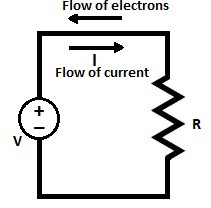Search

# What is Resistance?Definition and unit of Resistance

When voltage is applied to material, the flow of electrons through the material takes place. The number of electrons passing through the material varies and it depends on the electrical conductivity of the material. The metals have lesser resistance towards flow of electrons compared to insulating material. The electrons in the metals are loosely bound and it gets released easily with application of low voltage. In an insulator, these are tightly bound and a lot of energy is required to release the electrons.

When voltage is applied, the free electrons in the metal starts moving from lower potential to higher potential, and during drifting motion, electrons collide with each other and with the atom of the substance. This collision cause hindrance in the free movement of electrons. The impediment in the flow of electrons cause resistance.

Thus, the resistance can be defined as a property of the material which oppose the flow of electrons. The flow of current is just opposite to flow of electrons. In other words, we can say material property which oppose the flow of electric current is called the resistance.Resistance of the material is measured in Ohm. The symbol of the resistance is denoted by Greek letter(Ω). The symbol Ohms are named after Georg Simon Ohm (1784-1854), a German physicist who formulated Ohm's law after studying relationship between voltage ,current and resistance. The metals like silver,gold,aluminum and copper are good conductor of electricity because they offer less resistance. The insulator like rubber,paper and glass are bad conductor of electricity because of higher resistance. The lower the resistance, the higher the current flow and, the higher the resistance lower the current flow. The current flowing through the material is inversely proportion to its resistance.

## Unit of Resistance

The unit of resistance is Ohm(Ω). According to Ohm's Law;

R = V/I

When 1 volt is applied to circuit cause 1 ampere current to flow in the circuit, then the resistance of the circuit is 1Ω.

R = V/I = 1/1 = 1Ω

The resistance can also be expressed in the lower and bigger units of Ohm. These are milli-ohm,micro-ohm,kilo-ohm,mega-ohm,giga-ohm and tera-ohm.Resistance of different material is unlike and its depends upon the shape and the material property.

## Resistance of Different Materials

The materials are divided into three categories depending on the resistance value.

1. The metals like silver,gold,aluminum and copper offer very low resistance and are good conductor of electricity. The silver is the best conductor but it is not used because of its higher cost. Aluminum is widely used as a conductor because of its lower cost and availability.For transmission line aluminum is used because of its lower specific weight. Copper is mainly used for winding of the electrical machines and for electric bus bars. The copper is costlier than aluminum.

2. The other class of material is semiconductor. The semiconductor has higher resistance than conductor and lower resistance than insulator. The semiconductor material is widely used in electronics circuit, power electronics and communication circuits.

3. The third category of material is insulator. Insulating material have very high resistance and these materials like paper, wood,glass, PVC, XLPE,SF6, Vacuum etc are bad conductor of electricity. The insulators are used in electrical network to impede the flow of electric current.

## Effect of Temperature on Resistance

The different materials conductor, semiconductor and insulator have different resistance behavior with increased temperature.

The resistance of metals increased with an increase in the temperature. With increased temperature the collisions of the electrons with the atom increase and if offers more resistance at higher temperature. The conducting material has positive temperature coefficient of resistance. The losses in the conductor increase with increase in temperature.

The resistance of the semiconductor material decrease with an increase in the temperature. At increased temperature the numbers of free carriers increase and as a result the resistance gets decreased. The semiconductor material has negative temperature coefficient of resistance. The reliable working of the semiconductor devices can be ensured by maintaining the lower surrounding temperature.

The resistance of the insulating material decrease with increase in temperature.The insulating material has negative temperature coefficient of resistance.